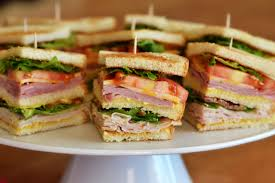# GMAT & MBA Admissions Blogin this series, one of our most experienced GMAT tutors, John Easter, applies useful strategies to answer questions from the 2018 Official Guide.

Problem #167 of the 2018 Official Guide to the GMAT states that four extra-large sandwiches of exactly the same size were ordered for m students where m>4. Three of the sandwiches were evenly divided among the students. Since 4 students did not want any of the fourth sandwich, it was evenly divided among the remaining students. If Carol ate one piece from each of the four sandwiches, the amount of sandwich that she ate would be what fraction of a whole extra-large sandwich?

(A) (m+4)/(m(m-4)

(B) (2m-4)/(m(m-4))

(C) (4m-4)/(m(m-4))

(D) (4m-8)/(m(m-4))

(E) (4m-12)/(m(m-4))

It's a VIC (variables in choices), and there is only one variable, so, obviously, it's time to back-solve!

STEP 1: Pick a nice value for m that is greater than 4. This is our pick.

Anything should work, so let's say m = 6.

STEP 2: Solve the problem when m = 6. The result is our target number.

So, six students divided three sandwiches equally. That means each of these students got1/6 of each of three sandwiches. Now, because 4 students didn't want any of the last sandwich is was divided equally between the other 2 students, one of whom was Carol. So, Carol ate 1/6 of each of the three sandwiches that everyone shared and1/2 of the forth sandwich.

Carol ate 1/6 + 1/6 + 1/6 + 1/2 sandwiches. That's 1 sandwich. So, our target number is 1.

STEP 3: Plug our pick into the answer choices until we find our target number.

A nice thing to notice is that all of our answers have the same denominator: m(m-4). If we plug 6 into that denominator we get 12. Since our target number is 1, we only need to find an answer with a numerator equal to 12.

(A) 6 + 4 = 10. Nope

(B) 2(6) - 4 = 8. Nope

(C) 4(6) - 4 = 20. Nope

(D) 4(6) - 8 = 16. Nope

(E) 4(6) - 12 = 12. Yep